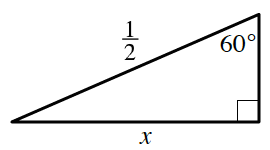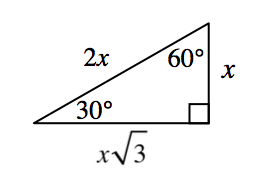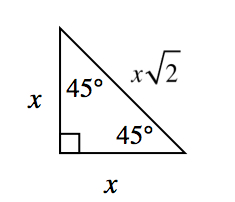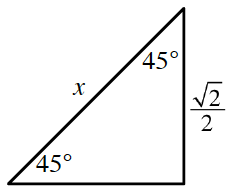### Home > INT3 > Chapter 8 > Lesson 8.3.2 > Problem8-117

8-117.

What is the length of the side labeled $x$ in each diagram?

1.This is a special right triangle.
You can leave the answer in fraction form.1.Use the same method from part (a).

$x = 1$